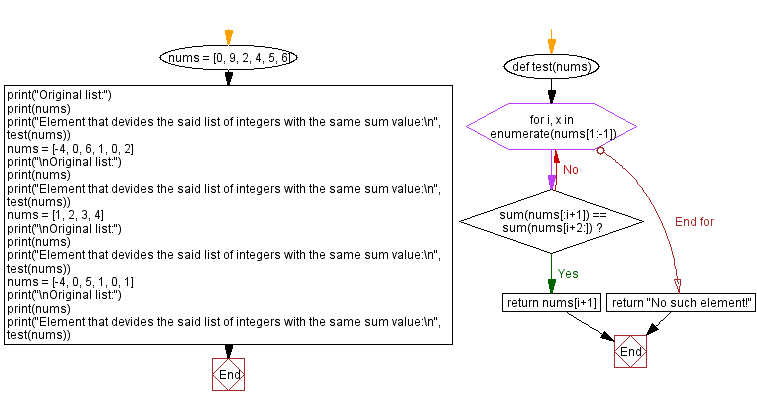﻿ Python: Divide a list of integers with the same sum value

# Python Exercises: Divide a list of integers with the same sum value

## Python List: Exercise - 273 with Solution

Write a Python program to find an element that divides a given list of integers with the same sum value.

Sample Solution-1:

Python Code:

``````# Define a function named 'test' that takes a list of integers 'nums' as input.
def test(nums):
# Iterate through the elements of 'nums' using 'enumerate'.
for i, x in enumerate(nums[1:-1]):
# Check if the sum of elements on the left of 'x' (before the current index)
# is equal to the sum of elements on the right of 'x' (after the next index).
if sum(nums[:i+1]) == sum(nums[i+2:]):
# If the condition is met, return the element 'x'.
return nums[i+1]
# If no such element is found, return a message indicating that there's no such element.
return "No such element!"

# Define a list of integers 'nums'.
nums = [0, 9, 2, 4, 5, 6]
print("Original list:")
print(nums)
# Call the 'test' function to find an element that divides the list with the same sum value on both sides.
print("Element that divides the list with the same sum value:\n", test(nums))

# Define another list of integers 'nums'.
nums = [-4, 0, 6, 1, 0, 2]
print("\nOriginal list:")
print(nums)
# Call the 'test' function for the second list.
print("Element that divides the list with the same sum value:\n", test(nums))

# Define one more list of integers 'nums'.
nums = [1, 2, 3, 4]
print("\nOriginal list:")
print(nums)
# Call the 'test' function for the third list.
print("Element that divides the list with the same sum value:\n", test(nums))

# Define an additional list of integers 'nums'.
nums = [-4, 0, 5, 1, 0, 1]
print("\nOriginal list:")
print(nums)
# Call the 'test' function for the last list.
print("Element that divides the list with the same sum value:\n", test(nums))
```
```

Sample Output:

```Original list:
[0, 9, 2, 4, 5, 6]
Element that devides the said list of integers with the same sum value:
4

Original list:
[-4, 0, 6, 1, 0, 2]
Element that devides the said list of integers with the same sum value:
1

Original list:
[1, 2, 3, 4]
Element that devides the said list of integers with the same sum value:
No such element!

Original list:
[-4, 0, 5, 1, 0, 1]
Element that devides the said list of integers with the same sum value:
1
```

Flowchart:Sample Solution-2:

Python Code:

``````# Define a function named 'test' that takes a list of integers 'nums' as input.
def test(nums):
# Iterate through the indices of 'nums'.
for i in range(len(nums)):
# Check if the sum of elements from the beginning of 'nums' up to index 'i'
# is equal to the sum of elements from index 'i' to the end of 'nums'.
if sum(nums[0:i+1]) == sum(nums[i:]):
# If the condition is met, return the element at index 'i'.
return nums[i]
# If no such element is found, return a message indicating that there's no such element.
return "No such element!"

# Define a list of integers 'nums'.
nums = [0, 9, 2, 4, 5, 6]
print("Original list:")
print(nums)
# Call the 'test' function to find an element that divides the list with the same sum value on both sides.
print("Element that divides the list with the same sum value:\n", test(nums))

# Define another list of integers 'nums'.
nums = [-4, 0, 6, 1, 0, 2]
print("\nOriginal list:")
print(nums)
# Call the 'test' function for the second list.
print("Element that divides the list with the same sum value:\n", test(nums))

# Define one more list of integers 'nums'.
nums = [1, 2, 3, 4]
print("\nOriginal list:")
print(nums)
# Call the 'test' function for the third list.
print("Element that divides the list with the same sum value:\n", test(nums))

# Define an additional list of integers 'nums'.
nums = [-4, 0, 5, 1, 0, 1]
print("\nOriginal list:")
print(nums)
# Call the 'test' function for the last list.
print("Element that divides the list with the same sum value:\n", test(nums))
```
```

Sample Output:

```Original list:
[0, 9, 2, 4, 5, 6]
Element that devides the said list of integers with the same sum value:
4

Original list:
[-4, 0, 6, 1, 0, 2]
Element that devides the said list of integers with the same sum value:
1

Original list:
[1, 2, 3, 4]
Element that devides the said list of integers with the same sum value:
No such element!

Original list:
[-4, 0, 5, 1, 0, 1]
Element that devides the said list of integers with the same sum value:
1
```

Flowchart:Python Code Editor:

Previous Python Exercise: Generates a list of numbers in the arithmetic progression within a range.
Next Python Exercise: Count lowercase letters in a list of words.

What is the difficulty level of this exercise?

Test your Programming skills with w3resource's quiz.

﻿